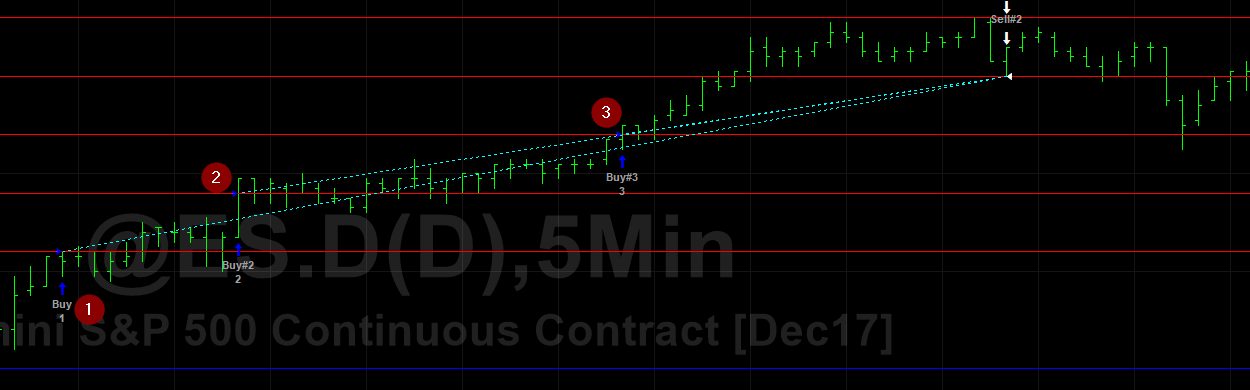# Long Entry Logic and EasyLanguage Code for Pyramiding Algorithm

Sorry for the delay in getting this up on the web.  Here is the flip side of the pyramiding day trade scheme from the buy side perspective.  I simply flipped the rules.  In some cases, to keep the programming a little cleaner I like to keep the buy and sellShort logic in two separate strategies.  So with this chart make sure you insert both strategies.And here is the code:

``````vars: mp(0),lastTradePrice(0),canBuy(true);

mp = marketPosition * currentContracts;

if date <> date then
begin
end;

if mp = 1 then canBuy = false;
if time > 1430 then canBuy = false;

if mp = 0 and canBuy = true then buy next bar at OpenD(0) + 3 stop;
if mp = 1 then buy next bar at OpenD(0) + 6 stop;
if mp = 2 then buy next bar at OpenD(0) + 9 stop;

if mp = 1 then lastTradePrice = OpenD(0) + 3;
if mp = 2 then lastTradePrice = OpenD(0) + 6;
if mp = 3 then lastTradePrice = OpenD(0) + 9;

if mp <> 0 then sell next bar at lastTradePrice - 3 stop;

if mp = 3 and barsSinceEntry > 0 and highD(0) > lastTradePrice + 3 then sell next bar at highD(0) - 3 stop;

setExitOnClose;``````
Pyramiding 3 Handles Up and Trailing Stop